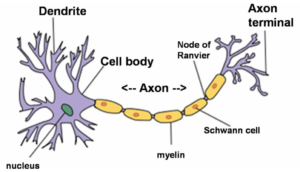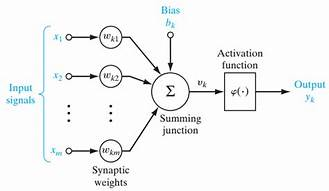# Deep Learning-Basics

## Perceptron an artificial Neuron

A brain is composed of cells called neurons that process information and connections between neurons called synapses through which information is transmitted. It is estimated that the human brain is approximately composed of 100 billion neurons and 100 trillion synapses. The following image,
represents the main components of a neuron are dendrites, a body, and an axon.The dendrites receive electrical signals from other neurons. The signals are processed in the neuron’s body, which then sends a signal through the axon to another neuron.

A Perceptron functions analogously to a neuron, it accepts one or more inputs, processes them, and returns an output. The following figure shows a diagram of the Perceptron.#### Input Layer

Like Regression a perceptron has weights and bias, both will be input. For example, we may have three input weights and one bias. Weights are often initialized to small random values, such as values in the range 0 to 0.3, although more complex initialization schemes can be used. Like linear regression, larger weights indicate increased complexity and fragility of the model.

Note: It is desirable to keep weights in the network small and regularization techniques can be used.

The output, that is, the signal whereby the neuron transmits its activity outside is calculated by applying the activation function, also called the transfer function, to the weighted sum of the inputs. These functions have a dynamic between -1 and 1, or between 0 and 1.

So, if we take it mathematically, we can see that

y=mx+c

Where y is output, m is slope/weight and c is biased coefficient.

This mx+c is a linear function, which is called an activation or transfer function.

Now, let us understand how many neurons a species have

The number of neurons present in species

• Fruit fly: 100 thousand neurons
• Cockroach: One million neurons
• Mouse: 75 million neurons
• Cat: One billion neurons
• Dolphin: 19 to 23 billions
• Chimpanzee: 7 billion neurons
• Elephant: 23 billion neurons
• Human: 100 billion neurons

Neuroplasticity, also known as brain plasticity, neuroelasticity, or neural plasticity, is the ability of the brain to change continuously throughout an individual’s life, e.g., brain activity associated with a given function can be transferred to a different location, the proportion of grey matter can change, and synapses may strengthen or weaken over time. in other words its ability to change and adapt as a result of experience. When people say that the brain possesses plasticity, they are not suggesting that the brain is similar to plastic.

The first few years of a child’s life are a time of rapid brain growth. At birth, every neuron in the cerebral cortex has an estimated 2,500 synapses; by the age of three, this number has grown to a whopping 15,000 synapses per neuron.

Average Adult has about half that number of synapses. This is because as we gain new experiences, some connections are strengthened while others are eliminated. This process is known as synaptic pruning. Neurons that are used frequently develop stronger connections and those that are rarely or never used eventually die. By developing new connections and pruning away weak ones, the brain is able to adapt to the changing environment.

The average adult, however, has about half that number of synapses. Why? Because as we gain new experiences, some connections are strengthened while others are eliminated.

Now, let’s talk about Artificial Neural Network(ANN), as a single neuron cannot take a good decision, so we use thousands of neurons arranged in a layer by layer order where neurons in a layer are not connected but they connected with neurons of another layer. There are three basic layers

1-Input layer: having the same number of neurons as in input data.

2-Hidden Layer: Any layer between the input and output layer is called a hidden layer. It can have any number of layers and any number of neurons.

3-Output Layer: The last layer, it’s having the same number of neurons as the number of output. In regression it’s one, in binary classification it’s 2 and so on…

#### Activation

There is a set of activation functions that differs in complexity and output:

• Step function: This fixes the threshold value x (for example, x= 10). The function will return 0 or 1 if the mathematical sum of the inputs is at, above, or below the threshold value.
• Linear combination: Instead of managing a threshold value, the weighted sum of the input values is subtracted from a default value; we will have a binary outcome, but it will be expressed by a positive (+b) or negative (-b) output of the subtraction.
• Sigmoid: This produces a sigmoid curve, a curve having an S trend. Often, the sigmoid function refers to a special case of the logistic function.Logistic FunctionSigmoid Function

Programitically, we can achieve this by:

```from sklearn.datasets import fetch_20newsgroups
from sklearn.metrics import f1_score, classification_report
from sklearn.linear_model import Perceptron

categories = ['rec.sport.hockey', 'rec.sport.baseball', 'rec.autos']
newsgroups_train = fetch_20newsgroups(subset='train',categories=categories, remove=('headers', 'footers', 'quotes'))

newsgroups_test = fetch_20newsgroups(subset='test',
vectorizer = TfidfVectorizer()

X_train = vectorizer.fit_transform(newsgroups_train.data)
X_test = vectorizer.transform(newsgroups_test.data)
classifier = Perceptron(n_iter=100, eta0=0.1)
classifier.fit(X_train, newsgroups_train.target )
predictions = classifier.predict(X_test)
print(classification_report(newsgroups_test.target, predictions))```

output:

```             precision    recall  f1-score   support

0       0.85      0.92      0.89       396
1       0.85      0.81      0.83       397
2       0.89      0.86      0.87       399

avg / total       0.86      0.86      0.86      1192```

•Amit Kumar verma